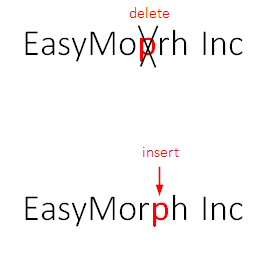## Aug 2, 2017

### Fuzzy matching and merging

Sometimes there are cases when it's hard to match (or merge) two tables because the matched values (e.g. names) are not precisely identical -- for instance when a name has a typo, or extra space between first and last name. Most frequently it happens when one of the tables is a result of a process that involved manual data entry at some point. It could be web-site forms, CRM systems, or spreadsheets. In such cases fuzzy matching comes to rescue. This article explains what this is and how to do it in EasyMorph.

Fuzzy matching can reliably match two words (or in a broader sense, text strings) that are similar but not exactly the same. For instance, "EasyMorph Inc" and "EasyMoprh Inc" clearly mean the same company, but the two names are not identical because the latter has a typo and therefore they won't be matched when doing a VLOOKUP in Excel, or data blending in Tableau.

In order to deal with such discrepancies the edit distance between two text strings can be calculated. This distance shows the number of edits required in one text in order to obtain the other.

The Levenshtein distance is the number of inserts, deletions and character replacements required to produce the other word. In our case, the Levenshtein distance between "EasyMoprh Inc" and "EasyMorph Inc" equals 2, because it requires removing "p" before "r" and inserting it after "r" -- so 2 edits in total.Levenshtein

There is a modified variant of the Levenshtein distance which is called the Damerau-Levenshtein distance. This variant adds swapping two adjacent characters as one more possible edit operation. The Damerau-Levenshtein distance between "EasyMoprh Inc" and "EasyMorph Inc" equals 1 because it only requires swapping "p" and "r" which is 1 edit operation.Damerau-Levenshtein

When fuzzy matching is done using the edit distance two text strings are considered matched when the distance is less than certain threshold (typically 2 or 3). The higher the threshold, the "fuzzier" the matching becomes. Therefore high thresholds are usually not practical as they produce too many false matches. Also, the shorter are matched values, the lower the threshold should be. For instance, the edit distance between "cat" and "dog" is just 3, but these are completely different words.

In EasyMorph, fuzzy matching is arranged using the "Match" transformation in the "Fuzzy" mode. It calculates the Damerau-Levenshtein distance for text values in two tables.

The transformation matches text values in one column (with mistyped values) in current table with a column in another table (with correct values). When the edit distance is less than or equal to the specified threshold values are considered matched. If merging other columns is required it can be done using additionally the "Merge" transformation by linking successfully matched values.

It is also possible to calculate the edit distance in expressions using distance(). The function can be used in simple cases cases when there is only one or two correct values to match with, thus avoiding creating a lookup table.